#### You may also like### Ball Bearings

If a is the radius of the axle, b the radius of each ball-bearing, and c the radius of the hub, why does the number of ball bearings n determine the ratio c/a? Find a formula for c/a in terms of n.### Far Horizon

An observer is on top of a lighthouse. How far from the foot of the lighthouse is the horizon that the observer can see?### Air Routes

Find the distance of the shortest air route at an altitude of 6000 metres between London and Cape Town given the latitudes and longitudes. A simple application of scalar products of vectors.

# What's That Graph?

##### Age 14 to 18Challenge Level

Elliot from Wilson's School sent the following explanations: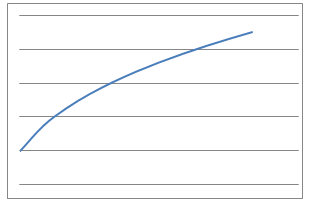The radius-number of pumps graph of the balloon links to the 1st graph, as the radius would gradually increase by less as the volume increases.The temperature-time graph for the tea links to the 2nd graph, as the temperature would decrease less as it cools down.The odometer reading-time graph links to the 3rd graph, as the car is moving at a constant speed, so the distance would also increase constantly.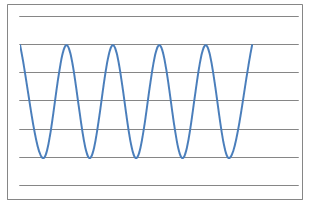The height-distance graph for the bicycle valve links with the 4th graph, as the valve's height would increase and decrease as the wheel rotates around.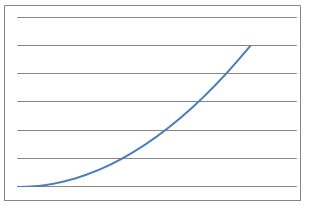The distance fallen-time graph links to graph 5, as you would accelerate, meaning your distance fallen would gradually increase by more.The volume-time graph for the water links to graph 6, as the water is sucked out at a constant rate, so the volume would decrease at a constant rate.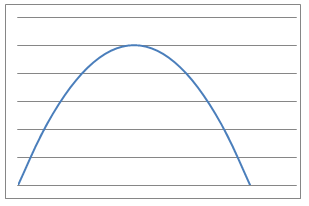The height-time graph for the tennis ball would be graph 7, as the height would increase when you throw it, then gradually stop increasing and decrease again as it falls.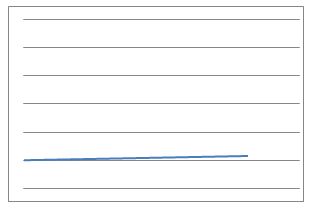The metres-inches graph links to graph 8, as the number of inches would be much greater than the number of metres, creating a very shallow straight line.

Niharika came up with some processes of her own that each graph could represent.
Linden explained the shape of each graph and found the correct equations.